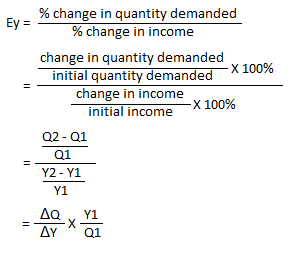# Measuring Income Elasticity of Demand: Percentage, Point and Arc Methods

Income elasticity of demand is the measure of degree of change in quantity demanded for a commodity in response to the change in income of the consumers demanding the commodity.

In simple words, it can be defined as the change in demand as a result of change in income of the consumers. Often referred to as just ‘income elasticity’, it is denoted by Ey.

Consumer’s income is one of the major factors that determine demand of a product.

Unlike price of the product, consumer’s income share direct relationship with the demand for the product. This implies that higher the income, more will be the demand, and lower the income, fewer will be the demand of the commodity.

### Methods of Measuring Income Elasticity of Demand

Basically, there are three methods by which we can measure income elasticity of demand. These methods are

i. Percentage method

ii. Point method

iii. Arc method

### Percentage Method

Percentage method is one of the commonly used approaches of measuring income elasticity of demand, under which income elasticity is measured in terms of rate of percentage change in quantity demanded of the commodity to percentage change in income of the consumers who demand that commodity.

According to this method, income elasticity can be mathematically expressed asWhere,

ΔQ = change in quantity demanded = Q2 – Q1

Q1 = initial quantity demanded

Q2 = new quantity demanded

ΔY = change in income of the consumers = Y2 – Y1

Y1 = initial income of the consumers

Y2 = new income of the consumers

For example: The demand of quantity when the income of the consumer was Rs 3000 was 30 units. When his income increased by Rs 2000, the quantity of commodity demanded by him became 50 units. Here, income elasticity of demand can be calculated asSince Ey = 1, this is an example of unitary income elasticity of demand where percentage change in income of consumer is equal to percentage change in demand of the commodity.

### Point Method

Point method is one of the geometric methods of measuring income elasticity of demand at any given point on the income demand curve.
Income demand curve is an upward sloping curve in case of normal goods and a downward sloping curve in case of inferior goods.

However, the method of calculating income elasticity depends upon the nature of the income demand curve. These methods are described below.

### Price elasticity on a linear income demand curve

Figure: income demand curveFrom percentage method, we have known thatΔBAC and ΔAEQ1 are similar triangles in account of AAA property. Thus, ratios of the sides of both the triangles are equal.

This implies,Now, substituting equation (iii) in equation (ii),### Price elasticity on a non-linear income demand curve

If the income demand curve is of a non-linear nature, then income elasticity can be calculated by drawing a tangent at the point where income elasticity is to be known. Then income elasticity can be simply calculated by applying the equation (iv) given above.In the figure given above, we can see DD is a non-linear demand curve and P is the point whose income elasticity is to be calculated. Thus, a tangent MN is drawn through the point P to X-axis. Then income elasticity is simply calculated as### Arc Method

Arc method is also a geometric method of measuring income elasticity of demand between any two points on an income demand curve. While ‘point method’ is used to calculate income elasticity at any given point on an income demand curve, this method is used to measure income elasticity over a certain range or between two points on the curve.In the figure, we can see that AB is an arc on the income demand curve DD, and C is the mid-point of AB. Here, income elasticity of demand at point C is calculated by following ways.

At first, average of income as well as quantity demanded is measured.Then income elasticity is calculated by applying the formulaWhere,

ΔQ = change in quantity demanded = Q2 – Q1

Q1 = initial quantity demanded

Q2 = new quantity demanded

ΔY = change in income of consumer = Y2 – Y1

Y1 = initial income of consumer

Y2 = new income of consumer